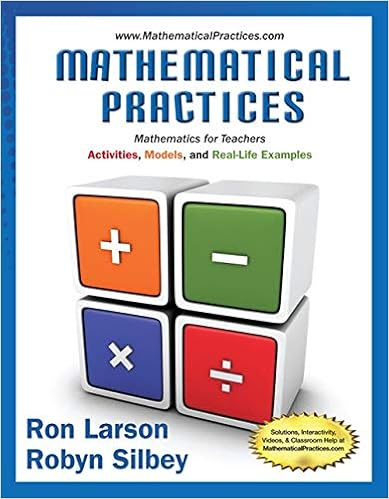# 84487 - Geometry Writing Assignment Introduction to...

• 3

This preview shows page 1 - 3 out of 3 pages.

##### We have textbook solutions for you!
The document you are viewing contains questions related to this textbook.The document you are viewing contains questions related to this textbook.
Chapter 10 / Exercise 31
Mathematical Practices, Mathematics for Teachers: Activities, Models, and Real-Life Examples
LarsonExpert Verified
Geometry Writing Assignment – Introduction to Triangles Each problem is worth 5 points Total Points: 50 Solve the problems pertaining triangles. Be sure to show all work when needed. 1. a. Based on the figure, Jordan says that RURW32. Kendra disagrees. Is either of them correct? Explain your reasoning. b. If QW = 27 inches, find QT. 2. Jordan says that from the information supplied in the diagram, she can conclude that R is on the perpendicular bisector of QS. Robert disagrees. Is either of them correct? Explain your reasoning.
##### We have textbook solutions for you!
The document you are viewing contains questions related to this textbook.The document you are viewing contains questions related to this textbook.
Chapter 10 / Exercise 31
Mathematical Practices, Mathematics for Teachers: Activities, Models, and Real-Life Examples
LarsonExpert Verified
3. Franklin measured and labeled the angles of a triangle as shown. Jennifer says that at least one of his measures is incorrect. Explain in at least two different ways how Jennifer knows that this is true. 4. Joey says that triangle XYZ is obtuse. Robin disagrees because there are more acute angles then
•••